TitleCollege Algebra
Tutorial 54D: Geometric Sequences and
SeriesLearning Objectives

 After completing this tutorial, you should be able to: Know what a geometric sequence is. Find the nth term of a geometric sequence. Write the formula for the nth term of a geometric sequence. Calculate a finite geometric series.Introduction

 In this tutorial we will mainly be going over geometric sequences and series.  We will be going forwards and backwards with this.  First we will be given the formula for the nth term and we will be finding specified terms.  Then we will turn it around and look at the terms and find the formula for the nth term.  We will finish up by looking at geometric series.  If you need a review on sequences, feel free to go to Tutorial 54A: Sequences.  If you need a review on sequences, feel free to go to Tutorial 54B: Series.  I think that you are ready to move ahead.Tutorial

 Geometric Sequence

 A geometric sequence is a sequence such that each successive term is obtained from the previous term by multiplying by a fixed number called a common ratio. The sequence 5, 10, 20, 40, 80, .... is an example of a geometric sequence.  The pattern is that we are always multiplying by a fixed number of 2 to the previous term to get to the next term. Be careful that you don't think that every sequence that has a pattern in multiplication is geometric. It is geometric if you are always multiplying by the SAME number each time. If you need a review on sequences, feel free to go to Tutorial 54A: Sequences.

 nth or General Term of a Geometric Sequence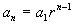where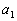is the first term of the sequence and r is the common ratio.Example 1:   Find the first five terms and the common ratio of the geometric sequence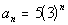.

 Since a geometric sequence is a sequence, you find the terms exactly the same way that you do a sequence.   n is our term number and we plug the term number into the function to find the value of the term. If you need a review on sequences, feel free to go to Tutorial 54A: Sequences. Lets see what we get for our first five terms: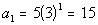*n = 1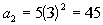*n = 2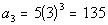*n = 3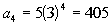*n = 4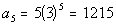*n = 5

 What would be the common ratio for this problem? If you said 3 you are correct!  Note that you would have to multiply by 3 to each previous term to get the next term:  (15)(3) = 45, (45)(3) = 135, (135)(3) = 405, and (405)(3) = 1215.   It has to be consistent throughout the sequence.  Also note that the base that is being raised to a power is 3.Example 2:   Find the first five terms and the common ratio of the geometric sequence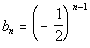.

 Since a geometric sequence is a sequence, you find the terms exactly the same way that you do a sequence.   n is our term number and we plug the term number into the function to find the value of the term. If you need a review on sequences, feel free to go to Tutorial 54A: Sequences. Lets see what we get for our first five terms:*n = 1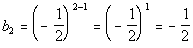*n = 2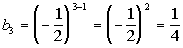*n = 3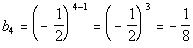*n = 4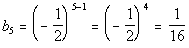*n = 5

 What would be the common ratio for this problem? If you said -1/2 you are correct!  Note that you would have to multiply by -1/2 each time you go from one term to the next: (1)(-1/2) = -1/2, (-1/2)(-1/2) = 1/4,  (1/4)(-1/2) = -1/8, and (-1/8)(-1/2)=1/16.   It has to be consistent throughout the sequence.  Also note that the base that is being raised to a power is -1/2.Example 3:   Write a formula for the nth term of the geometric sequence 7, 28, 112, 448, ....  Do not use a recursive formula.

 We will use the nth term formula for a geometric sequence,to help us with this problem. Basically we need to find two things: the first term of the sequence,and  the common ratio, r. What is, the first term? If you said 7, give yourself a high five.  The first term of this sequence is 7. What is r, the common ratio? If you said 4, you are right!!  Note that you would have to multiply 4 each time you go from one term to the next: (7)(4) = 28, (28)(4) = 112, and (112)(4) = 448.   It has to be consistent throughout the sequence. Putting in 7 forand 4 for r we get: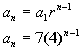Example 4:   Write a formula for the nth term of the geometric sequence 16, - 4, 1, -1/4, ....  Do not use a recursive formula.

 We will use the nth term formula for a geometric sequence,to help us with this problem. Basically we need to find two things: the first term of the sequence,and  the common ratio, r. What is, the first term? If you said 16, give yourself a high five.  The first term of this sequence is 16. What is r, the common ratio? If you said -1/4, you are right!!  Note that you would have to multiply -1/4 each time you go from one term to the next: (16)(-1/4) = - 4, (- 4)(-1/4) = 1, and (1)(-1/4) = -1/4.   It has to be consistent throughout the sequence. Putting in 16 forand -1/4 for r we get: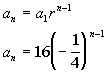Example 5:   Find the first term of a geometric sequence with a fifth term of 32 and a common ratio of -2.

 This problem has a little twist to it.  Now we are looking for the first term.  We can still use the nth term formula for a geometric sequence,,  to help us with this problem. We will just be looking for. Plugging in 32 for nth term, 5 for n,  and -2 for r we get: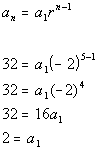The first term would have to be 2.Example 6:   Find the common ratio for a geometric sequence with a first term of 3/4 and a third term of 27/16.

 This problem has a little twist to it.  Now we are looking for the common ratio.  We can still use the nth term formula for a geometric sequence,,  to help us with this problem. We will just be looking for r. Plugging in 3/4 for, 3 for n,  and 27/16 for the nth term we get: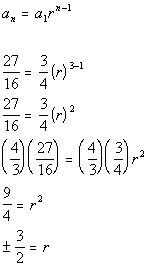The common ratio could be either 3/2 or -3/2.

 The Sum of the First n Terms of a Finite Geometric Sequence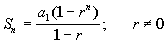is the first term of the sequence and r is the common ratio.

 If you need a review on series, feel free to go to Tutorial 54B: Series.Example 7:   Find the sum of the finite geometric series 3 - 6 + 12 - 24 + 48 - 96.

 We will use the formula for the sum of the first n terms of geometric sequence,, to help us with this problem. Basically we need to find three things: the first term of the sequence, the common ratio, and how many terms of the sequence we are adding in the series. What is, the first term? If you said 3 you are right! What is r, the common ratio? If you said -2, give yourself a pat on the back. Note that you would have to multiply -2 each time you go from one term to the next: (3)(-2) = - 6, (-6)(2) = -12, (-12)(-2) = 24, (24)(-2) = - 48, and (-48)(-4) = -96.   It has to be consistent throughout the sequence. How many terms are we summing up? If you said 6, you are correct. Putting in 3 for the first term, -2 for the common ratio, and 6 for n, we get: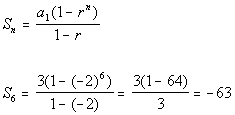Example 8:   Find the sum of the finite geometric series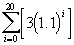.

 We will use the formula for the sum of the first n terms of geometric sequence,, to help us with this problem. Basically we need to find three things: the first term of the sequence, the common ratio, and how many terms of the sequence we are adding in the series. What is, the first term? If you said 3 you are right!   Since this summation starts at 0, you need to plug in 0 into the given formula: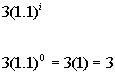What is r, the common ratio? If you said 1.1, give yourself a pat on the back.  Note that 1.1 is the number that is being raised to the exponent.  So each time the number goes up on the exponent, in essence you are multiplying it by 1.1 How many terms are we summing up? If you said 21, you are correct.  If you start at 0 and go all the way to 20, there will be 21 terms. Putting in 3 for the first term, 1.1 for the common ratio, and 21 for n, we get: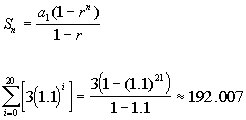The Sum of an Infinite Geometric Series If -1 < r < 1 (or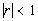), then the sum of the infinite geometric series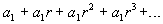in whichis the first term and r is the common ratio is given by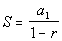. If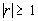, the infinite series does NOT have a sum.Example 9:   Find the sum of the infinite series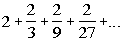, if possible.

 We will use the formula for the sum of infinite geometric sequence,,  to help us with this problem. Basically we need to find two things: the first term of the sequence and the common ratio. What is the first term,? If you said 2 you are right! What is the common ratio, r? If you said 1/3, give yourself a pat on the back. Note that you would have to multiply 1/3 each time you go from one term to the next: (2)(1/3) = 2/3, (2/3)(1/3) = 2/9, (2/9)(1/3) = 2/27.   It has to be consistent throughout the sequence. Putting in 2 for the first term and 1/3 for the common ratio we get: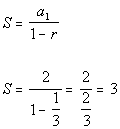Example 10:   Find the sum of the infinite series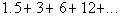, if possible.

 We will use the formula for the sum of infinite geometric sequence,,  to help us with this problem. Basically we need to find two things: the first term of the sequence and the common ratio. What is the first term,? If you said 1.5 you are right! What is the common ratio, r? If you said 2, give yourself a pat on the back. Note that you would have to multiply 2 each time you go from one term to the next: (1.5)(2) = 3, (3)(2) = 6, (6)(2) = 12.   It has to be consistent throughout the sequence. Since the geometric ratio is 2 and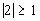, there is no sum.Example 11:   Find the sum of the infinite series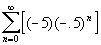, if possible.

 We will use the formula for the sum of infinite geometric sequence,,  to help us with this problem. Basically we need to find two things: the first term of the sequence and the common ratio. What is the first term,? If you said -5 you are right!   Since this summation starts at 0, you need to plug in 0 into the given formula: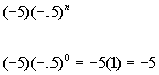What is the common ratio, r? If you said -.5, give yourself a pat on the back. Note that -.5 is the number that is being raised to the exponent.  So each time the number goes up on the exponent, in essence you are multiplying it by -.5. Putting in -5 for the first term and -.5 for the common ratio we get:Practice Problems

 These are practice problems to help bring you to the next level.  It will allow you to check and see if you have an understanding of these types of problems. Math works just like anything else, if you want to get good at it, then you need to practice it.  Even the best athletes and musicians had help along the way and lots of practice, practice, practice, to get good at their sport or instrument.  In fact there is no such thing as too much practice. To get the most out of these, you should work the problem out on your own and then check your answer by clicking on the link for the answer/discussion for that  problem.  At the link you will find the answer as well as any steps that went into finding that answer.Practice Problems 1a - 1b: Find the first five terms and the common ratio of the given geometric sequence.

 1a.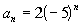(answer/discussion to 1a) 1b.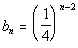(answer/discussion to 1b)Practice Problems 2a - 2b: Write a formula for the nth term of the given geometric sequence.  Do not use a recursive formula.

 2a. 125, 25, 5, 1, ... (answer/discussion to 2a) 2b. 4, -12, 36, -108, ... (answer/discussion to 2b)Practice Problems 3a - 3b: Find the sum of the given finite geometric series.

 3a. 2 + 14 + 98 + 686 + 4802 + 33614 + 235298 (answer/discussion to 3a) 3b.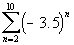(answer/discussion to 3b)Practice Problems 4a - 4b: Find the sum of the given infinite geometric series,
if possible.

 4a.(answer/discussion to 4a) 4b.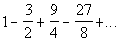(answer/discussion to 4b)Need Extra Help on these Topics?

 There were no good websites found to help us with the topics on this page.   Go to Get Help Outside the Classroom found in Tutorial 1: How to Succeed in a Math Class for some more suggestions.

Last revised on May 17, 2011 by Kim Seward.